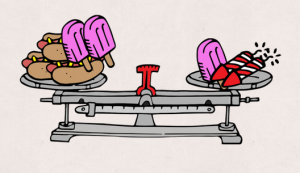Over Age 30 and Taking the GRE? Learn These 3 Key Things!   Get the FREE Video!

# Simultaneous Equations on the GRE

Earlier this month on my Facebook Page I posted this fun brain game (below) from American Mensa that asks you a seemingly straightforward question:

If the scales below are evenly balanced, how many fireworks must you use to replace the question mark in order to keep the scale on the right even?But of course it’s not quite as easy as it might seem at first blush.

If you haven’t tried your hand at this problem yet, going ahead and give it a whirl before reading any further. Whether you’ve tried to solve it yet or not, here’s a hint for you: Convert the middle scale into an equation and solve it first. It will help you figure out the other two!

In fact, that’s the crux of this problem and why it has direct applications to the types of questions you may encounter on the quantitative section of the GRE (albeit in not quite as fun of a format!). Specifically, think about simultaneous equations. Simultaneous equations are an advanced GRE algebra concept (covered in our Full GRE Course) that enable you to solve multiple equations with multiple variables. And that’s exactly what we have going on here, right?

First, convert each scale to an equation where hotdogs = x, popsicles = y, and fireworks = z:

Scale 1: 2x + 4y = 1x + 3z

Scale 2: 2x + 3y = 4x + 2y

Scale 3: 4x + 2y = 1y + ?z

Solving Scale 2 first for y, you get y = 2x. Replace all the y variables with 2x. Next, solve Scale 1 for z. You’ll get 3x  = z.

With one variable remaining, you can now solve for the question mark. The answer is:

? = 2

2 Fireworks are needed to balance the scale!Well done if you got that. Still confused? Leave your questions or comments below! And be sure to “Like” our Facebook Page for more GRE tips and sample questions.